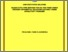# Runge-Kutta type methods for solving third-order ordinary differential equations and first-order oscillatory problems

## Citation

Alshareeda, Firas Adel Fawzi (2017) Runge-Kutta type methods for solving third-order ordinary differential equations and first-order oscillatory problems. Doctoral thesis, Universiti Putra Malaysia.

## Abstract

In this study, new Runge-Kutta type methods (RKTG) are derived for directly solving third-order ordinary differential equations ODEs of the formy′′′=f(x,y,y′). The derivation of third-, fourth- and fifth-order explicit RKTG methods using constant step length and an embedded explicit RKTG denoted by RKTGD methods of 4(3) and 5(4)pairs for variable step size have been derived. Numerical results obtained show that the new RKTG and RKTGD methods are more accurate and efficient than several existing methods in the literature. In the second part, a Runge-Kutta type methods are derived for directly solving general third-order differential equations of the formy′′′=f(x,y,y′,y′′)denoted as (RKTGG).The derivation of third- and fourth-order explicit RKTGG methods using constant step size have been derived. Numerical results obtained for the new methods have shown efficiency and robustness in terms of accuracy and number of function evaluations. Next, an explicit Runge-Kutta (RK) and modified Runge-Kutta (MRK) with dispersion and dissipation properties are studied for the integration of initial value problems (IVPs) of first-order ordinary differential equations (ODEs) possessing oscillating solutions. The constructions of RK and MRK methods for constant step size and embedded MRK pair for variable step length have been derived. The strategies in choosing the free parameters are also discussed. The impacts of dispersion and dissipation relations are tested on homogeneous and non-homogeneous test problems which have oscillating solutions. Meanwhile, trigonometrically-fitted RK and MRK methods are constructed for integration of IVPs of first-order ODEs with periodic behavior. The derivation of fourth-order trigonometrically-fitted explicit RK and MRK methods using constant step size and embedded 6(5) pair trigonometrically-fitted explicit Runge-Kutta (ETFRK) method for variable step size have been derived. We analyzed the numerical results of RK,MRK and ETFRK methods and found that our new methods are more efficient than the existing methods .In conclusion, the new codes developed are suitable for solving system of first-order ODEs in which the solutions are in the oscillatory form and third-order ODEs.Preview
Text
FS 2017 30 IR.pdfView Item